##### Differential Equations For DummiesLinear approximation is not only easy to do, but also very useful! For example, you can use it to approximate a cubed root without using a calculator.

Here's an example. Can you approximate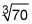Like this: Bingo! 4.125.

Well, okay, there's a little more to it than that. Take a look at the figure and then follow the steps below to get the full picture.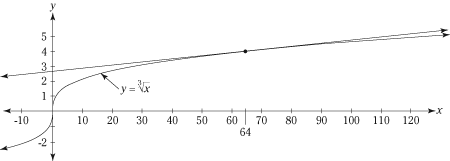The line tangent to the curve at (64, 4) can be used to approximate cube roots or numbers near 64.

To estimate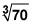1. Find a perfect cube root nearYou notice that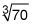is near a no-brainer,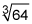which, of course, is 4. That gives you the point (64, 4) on the graph of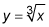2. Find the slope of(which is the slope of the tangent line) at x = 64.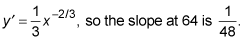This tells you that — to approximate cube roots near 64 — you add (or subtract)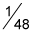to 4 for each increase (or decrease) of one from 64. For example, the cube root of 65 is about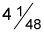the cube root of 66 is about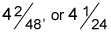the cube root of 67 is about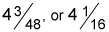and the cube root of 63 is about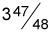3. Use the point-slope form to write the equation of the tangent line at (64, 4).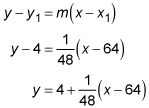In the third line of the above equation, you put the 4 in the front of the right side of the equation (instead of at the far right which might seem more natural) for two reasons. First, because doing so makes this equation jibe with the explanation at the end of Step 2 about starting at 4 and going up (or down) from there as you move away from the point of tangency. And second, to make this equation agree with the explanation at the end of Step 4. You'll see how it all works in a minute.

4. Because this tangent line runs so close to the function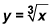near x = 64, you can use it to estimate cube roots of numbers near 64, like at x = 70.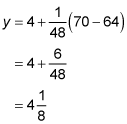By the way, in your calc text, the simple point-slope form from algebra (first equation line in Step 3) is probably rewritten in highfalutin' calculus terms — like this: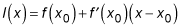Don't be intimidated by this equation. It's just your friendly old algebra equation in disguise! Look carefully at it term by term and you'll see that it's mathematically identical to the point-slope equation tweaked like this: y = y1 + m(xx1). (The different subscript numbers, 0 and 1, have no significance.)